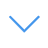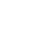# Geometry Fresh

Geometry Fresh is one of the Math Games that you can play on UGameZone.com for free. Exercise your math skills with this game! Can you see the pattern of the game? Find the corresponding figure of the number using the mathematical algorithm calculation, choose the correct answer!

Read More【Esc】を押して全画面表示を終了します

# Geometry Fresh

Geometry Fresh is one of the Math Games that you can play on UGameZone.com for free. Exercise your math skills with this game! Can you see the pattern of the game? Find the corresponding figure of the number using the mathematical algorithm calculation, choose the correct answer!You can use UGameZone Flash Enabler plugin to enable Flash automatically. Or you can follow our instructions to enable Flash manually.

play automatically

## Game Ratings

86%

781
162
Category
Math Games
タグ

Geometry Fresh is one of the Math Games that you can play on UGameZone.com for free. Exercise your math skills with this game! Can you see the pattern of the game? Find the corresponding figure of the number using the mathematical algorithm calculation, choose the correct answer!